# SAT II Math II : Analyzing Figures

## Example Questions

### Example Question #1 : Analyzing Figures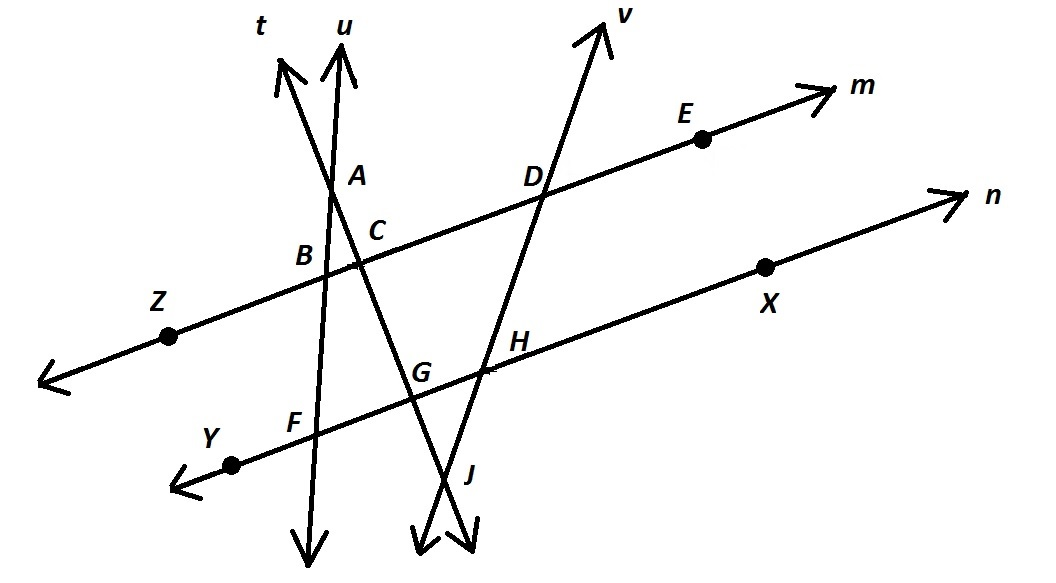Refer to the above diagram. Which of the following is not a valid name for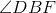?

All of the other choices give valid names for the angle.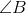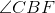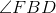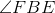Explanation:is the correct choice. A single letter - the vertex - can be used for an angle if and only if that angle is the only one with that vertex. This is not the case here. The three-letter names in the other choices all follow the convention of the middle letter being vertexand each of the other two letters being points on a different side of the angle.

### Example Question #2 : Analyzing Figures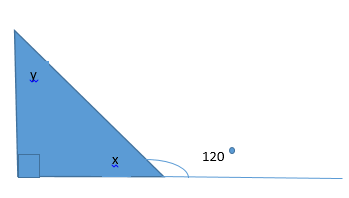Use the rules of triangles to solve for x and y.

x=30, y=30

x=30, y=60

x=45, y=45

x=60, y=30

x=60, y=30

Explanation:

Using the rules of triangles and lines we know that the degree of a straight line is 180. Knowing this we can find x by creating and solving the following equation: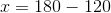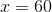Now using the fact that the interior angles of a triangle add to 180 we can create the following equation and solve for y: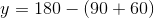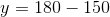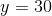### Example Question #3 : Analyzing Figures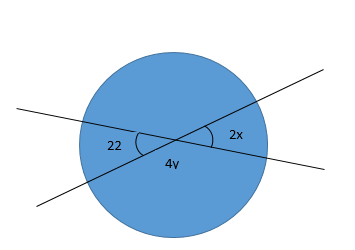Use the facts of circles to solve for x and y.

x=10, y=30

x=11, y= 39.5

x=39.5, y=11

x=13, y=10

x=11, y= 39.5

Explanation:

In this question we use the rule that oppisite angles are congruent and a line is 180 degrees. Knowing these two facts we can first solve for x then solve for y.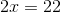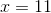Then: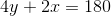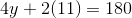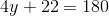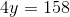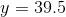### Example Question #4 : Analyzing Figures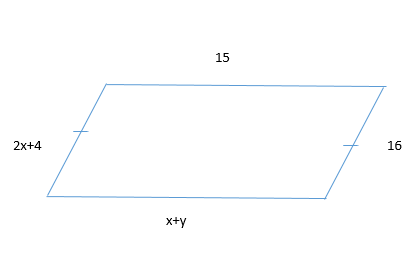Solve for x and y using the rules of quadrilateral

x=2, y=4

x=6, y=9

x=6, y=10

x=9, y=6

x=6, y=9

Explanation:

By using the rules of quadrilaterals we know that oppisite sides are congruent on a rhombus. Therefore, we set up an equation to solve for x. Then we will use that number and substitute it in for x and solve for y.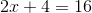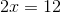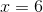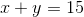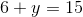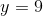### Example Question #5 : Analyzing Figures

Chords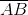andintersect at point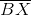is twice as long as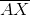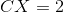and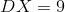Give the length of.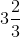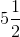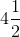Explanation:

If we let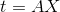, then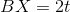The figure referenced is below (not drawn to scale):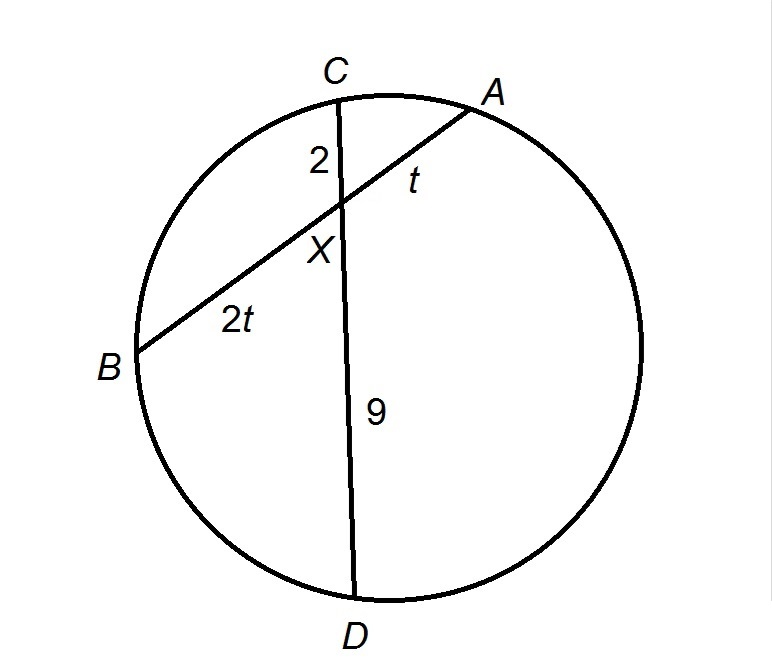If two chords intersect inside the circle, then the cut each other so that for each chord, the product of the lengths of the two parts is the same; in other words,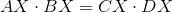Setting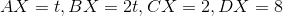, and solving for: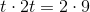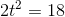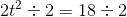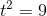Taking the positive square root of both sides: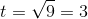,

the correct length of.